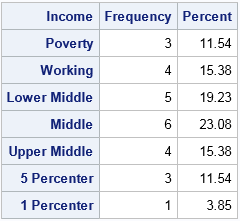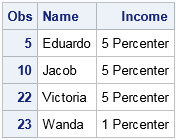SAS formats are flexible, dynamic, and have many uses. For example, you can use formats to count missing values and to change the order of a categorical variable in a table or plot. Did you know that you can also use SAS formats to recode a variable or to bin a numerical variable into categories? This can be very convenient because you do not need to create a new variable in the data set; you merely apply a format to an existing variable.

### Income categories: Are you middle class?

Many people use several IF-THEN/ELSE statements in the DATA step (or in a DATA step view) to create a new discrete variable that represents binned values of a continuous variable. That is a fine technique, but an alternative technique is to create a user-defined format that bins a continuous variable. One advantage of a custom format is that you can apply the format to multiple variables in multiple data sets.

For example, suppose that you want to define income categories such as "working class," "middle class," and the ultra-rich "1 percenters." According to a 2012 Money magazine article, the following cut points divide US household income into seven categories:

```/* 2012 income categories for US according to Money magazine */ proc format; value IncomeFmt low -< 23000 = "Poverty" /* < 23 thousand */ 23000 -< 32500 = "Working" /* [ 23, 32.5) thousand */ 32500 -< 60000 = "Lower Middle" /* [ 32.5, 60) thousand */ 60000 -< 100000 = "Middle" /* [ 60, 100) thousand */ 100000 -< 150000 = "Upper Middle" /* [100, 150) thousand */ 150000 -< 250000 = "5 Percenter" /* [150, 250) thousand */ 250000 - high = "1 Percenter"; /* > 250 thousand */ run;```

The call to PROC FORMAT creates a custom format called IncomeFmt. When you assign the IncomeFmt format to a numerical variable, SAS will look at the value of each observation and determine the formatted value from the raw value. For example, a value of 18,000 is less than 23,000, so that value is formatted as "Poverty." A value of 85,000 is in the half-open interval [60000, 100000), so that value is formatted as "Middle."

The following DATA step defines the incomes for 26 fictitious individuals. The IncomeFmt format is assigned to the Income variable:

```data incomes; length Name \$10.; input Name Income @@; format Income IncomeFmt.; /* assign IncomeFmt format to Income variable */ datalines; Amy 65100 Brad 146500 Carlos 113300 Dimtri 28800 Eduardo 233300 Felicity 14600 Guo 43400 Hector 141700 Irene 53400 Jacob 170300 Katerina 43100 Liu 66800 Michael 15800 Nancy 30900 Oscar 31800 Pablo 65800 Quentin 40000 Rick 62200 Stephan 32900 Tracy 64000 Umberto 124000 Victoria 220700 Wanda 263800 Xie 9300 Yolanda 23400 Zachary 93800 ;```

The Income variable is a continuous variable, but the format bins each value into one of seven discrete values. Consequently, SAS procedures can analyze the Income variable as if it were a discrete variable. For example, you can count the number of individuals in each income category by calling PROC FREQ:

```proc freq data=incomes; tables Income / nocum norow nocol; run;```### Assigning or unassigning formats at run time

The underlying data values are not lost. You can use a FORMAT statement in a SAS procedure to temporarily assign or unassign a format. If you remove the format, you can analyze the underlying raw data. For example, the following call to PROC UNIVARIATE analyzes the raw incomes:

```proc univariate data=incomes; format Income; /* remove the format for this analysis */ var Income; run;```

In a similar way, if you specify the Income variable on a CLASS statement in a regression procedures, the formatted values are used for the analysis. However, if you do NOT include it on the CLASS statement, then the variable is treated as a continuous variable and the unformatted values are used.

### Subset data by using formatted values

If you run PROC PRINT on the income data, it LOOKS like the Income variable is a character variable. Furthermore, it is analyzed like a character variable when used in some SAS procedures such as PROC FREQ. Consequently, you might forget that the Income variable is actually numeric. However, if you treat Income as a character variable in the DATA set or a WHERE clause, then SAS will report an error. For example, the following WHERE clause is incorrect:

```proc print data=incomes; where Income in ("5 Percenter", "1 Percenter"); /* WRONG: Income is numeric */ run;```
```ERROR: WHERE clause operator requires compatible variables.
```

SAS reports an error because the WHERE clause cannot compare the raw (numeric) values of the Income variable with elements of a set that contains two strings. When you see an error message like this, use PROC CONTENTS to investigate the attributes of the variable:

```ods select Position; proc contents data=incomes order=varnum; run;```The output from PROC CONTENTS informs you that the Income variable is numeric and displays the name of the format that is attached to it.

If you know the cutoff values that are used for the format, you could create a valid WHERE clause that uses numeric values: where Income GE 150000. However, usually it makes more sense to create a valid WHERE clause by using the PUT statement to apply the format to the raw data and compare formatted values:

```/* use formatted values to subset data */ proc print data=incomes; where put(Income, IncomeFmt.) in ("5 Percenter", "1 Percenter"); run;```You can use other DATA step functions when constructing WHERE clauses. A typical example is when a variable is a SAS date. For example, the Sashelp.Air data set contains a variable named Date. You can use the following WHERE clause to analyze the subset of data that corresponds to the month of January in years prior to 1955:

```proc print data=Sashelp.Air; where month(date)=1 and year(date)<1955; /* all January dates prior to 1955 */ run;```

### Summary

As shown in this article, SAS formats are very useful and flexible:

• You can use a custom format to bin a continuous variable into categories.
• Within a SAS procedure, you can temporarily assign or unassign a format to change the way that the data are analyzed.
• The WHERE clause looks at raw data values, so use the PUT function in a WHERE clause if you want to subset the data according to the formatted values.
ShareDistinguished Researcher in Computational Statistics

Rick Wicklin, PhD, is a distinguished researcher in computational statistics at SAS and is a principal developer of SAS/IML software. His areas of expertise include computational statistics, simulation, statistical graphics, and modern methods in statistical data analysis. Rick is author of the books Statistical Programming with SAS/IML Software and Simulating Data with SAS.

1.Good post on a very useful SAS feature Rick!

A benefit in using a user-defined format is simpler management of your business definitions. If you have your format definitions centralized, and a change is required due to business reasons; for example the bounds for middle income change, you only need to change your proc format code and not all your individual SAS IF-THEN/ELSE statements.

This approach ensures consistency across reports and analysis and gains business trust as the same definition is used rather than multiple static definitions scattered across code.

•Well said.

2.Chris Hemedinger on

Excellent tip! As Michelle said, placing the logic in the format instead of in your DATA step code will allow for easier changes and experimentation.

For time series data (dates, datetimes), SAS offers a number of built-in formats to help you to bin your data into discrete periods like day, month, week, day-of-week, year, and so on. Check my blog about dates and datetimes for some examples of how useful this is.

3.Peter Lancashire on

Another related use of formats is to colour output using ODS styles. For example studentised residuals may be colour coded by adding this to PROC PRINT:
.

VAR StudentResid / style(data)={color=white fontweight=bold background=stdres.};

.
The format looks like this:
.

PROC FORMAT;
value stdres
low - -4.00 = h00060ff
-4.00 - -3.50 = h00070ff
/* and so on */
+3.50 - +4.00 = h07870ff
+4.00 - high = h07860ff
;
RUN;